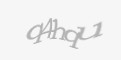# Object search in MSSQL 2005In query analyzer for Microsoft SQL 2000 you can search objects easily, just open Query Analyzer and press F4. In Microsoft SQL Server Management Studio for SQL 2005, this search functionality has been removed. (Microsoft said that nobody uses this function so it's useless), no comment for this reason. We need this functionality, so how to do it?

You need to use a script for searching in system objects. Basic script for all common objects is below.

```DECLARE @SEARCHSTRING VARCHAR(255), @notcontain Varchar(255)
SELECT @SEARCHSTRING = 'Text I am searching', @notcontain = ''
SELECT DISTINCT sysobjects.name AS [Object Name] ,
case
when sysobjects.xtype = 'C' then 'CHECK constraint'
when sysobjects.xtype = 'D' then 'Default or DEFAULT constraint'
when sysobjects.xtype = 'F' then 'Foreign Key'
when sysobjects.xtype = 'FN' then 'Scalar function'
when sysobjects.xtype = 'P' then 'Stored Procedure'
when sysobjects.xtype = 'PK' then 'PRIMARY KEY constraint'
when sysobjects.xtype = 'S' then 'System table'
when sysobjects.xtype = 'TF' then 'Function'
when sysobjects.xtype = 'TR' then 'Trigger'
when sysobjects.xtype = 'U' then 'User table'
when sysobjects.xtype = 'UQ' then 'UNIQUE constraint'
when sysobjects.xtype = 'V' then 'View'
when sysobjects.xtype = 'X' then 'Extended stored procedure'
end as [Object Type]
FROM sysobjects
WHERE
sysobjects.type in ('C','D','F','FN','P','K','S','TF','TR','U','V','X')
AND sysobjects.category = 0
AND CHARINDEX(@SEARCHSTRING,sysobjects.name)>0
AND ((CHARINDEX(@notcontain,sysobjects.name)=0 or
CHARINDEX(@notcontain,sysobjects.name)<>0))
```

@SEARCHSTRING is text in name we are searching. If we want exclude some text we can fill @notcontain variable.

For restriction on some requested objects we need to comment some parts of code, in WHEN part and change condition sysobjects.type in ('C','D','F','FN','P','K','S','TF','TR','U','V','X').

List of type objects, we can use:

```C = CHECK constraint
D = Default or DEFAULT constraint
F = FOREIGN KEY constraint
FN = Scalar function
IF = Inlined table-function
K = PRIMARY KEY or UNIQUE constraint
L = Log
P = Stored procedure
R = Rule
RF = Replication filter stored procedure
S = System table
TF = Table function
TR = Trigger
U = User table
V = View
X = Extended stored procedure
```
Author info
Author: Stanislav DubenWebsite: http://www.duben.org